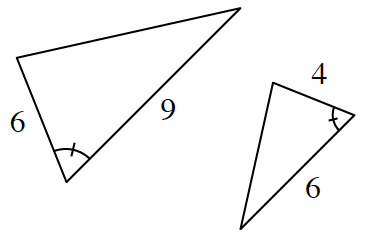### Home > CCG > Chapter Ch8 > Lesson 8.1.3 > Problem8-35

8-35.

Multiple Choice: Which property below can be used to prove that the triangles at right are similar?

1. $\text{AA}\sim$

1. $\text{SAS} \sim$

1. $\text{ SSS} \sim$

1. $\text {HL} \sim$

1. none of these(b) because there are two sets of proportional sides

$\left (\frac{4}{6}\approx 0.66 \text{ and }\frac{6}{9}\approx 0.66\right )$

with a congruent angle between them.